CBSE Class 10 Sample Paper for 2020 Boards - Maths Standard

Class 10
Solutions of Sample Papers for Class 10 Boards

## The angle of elevation of an airplane from point on the ground is 60°. After a flight of 30 seconds, the angle of elevation becomes 30°. If the airplane is flying at a constant height of 3000√3 m, find the speed of the airplane.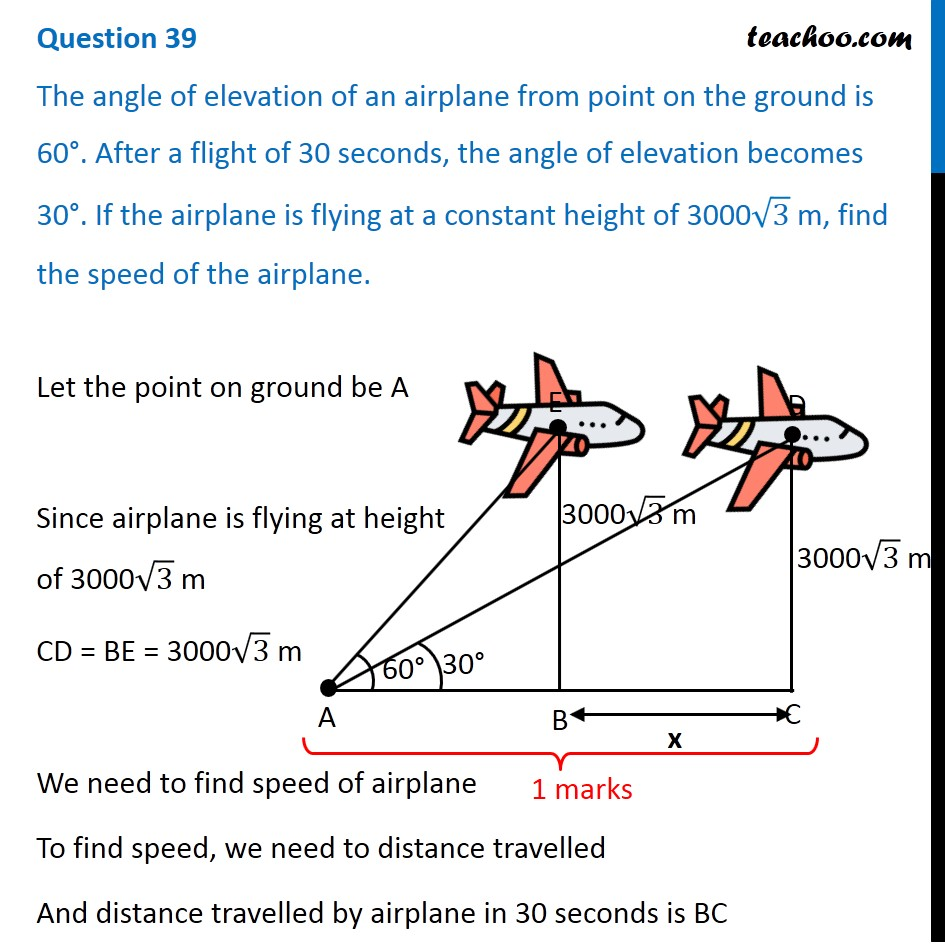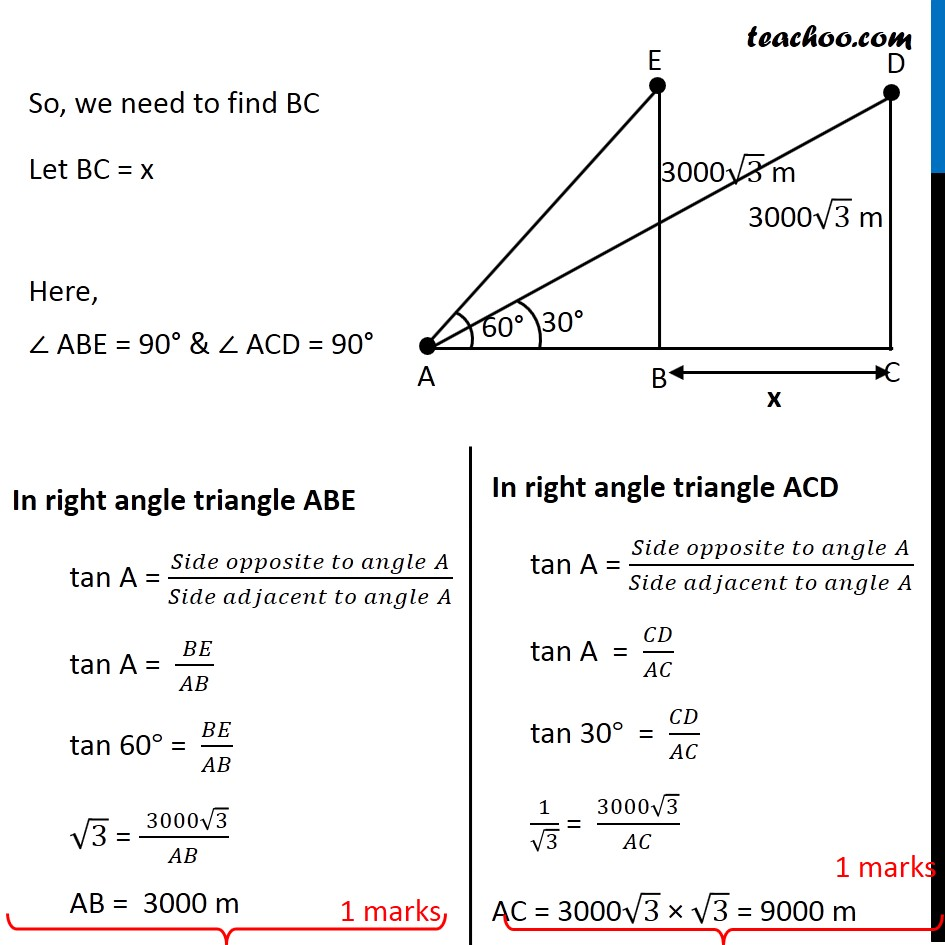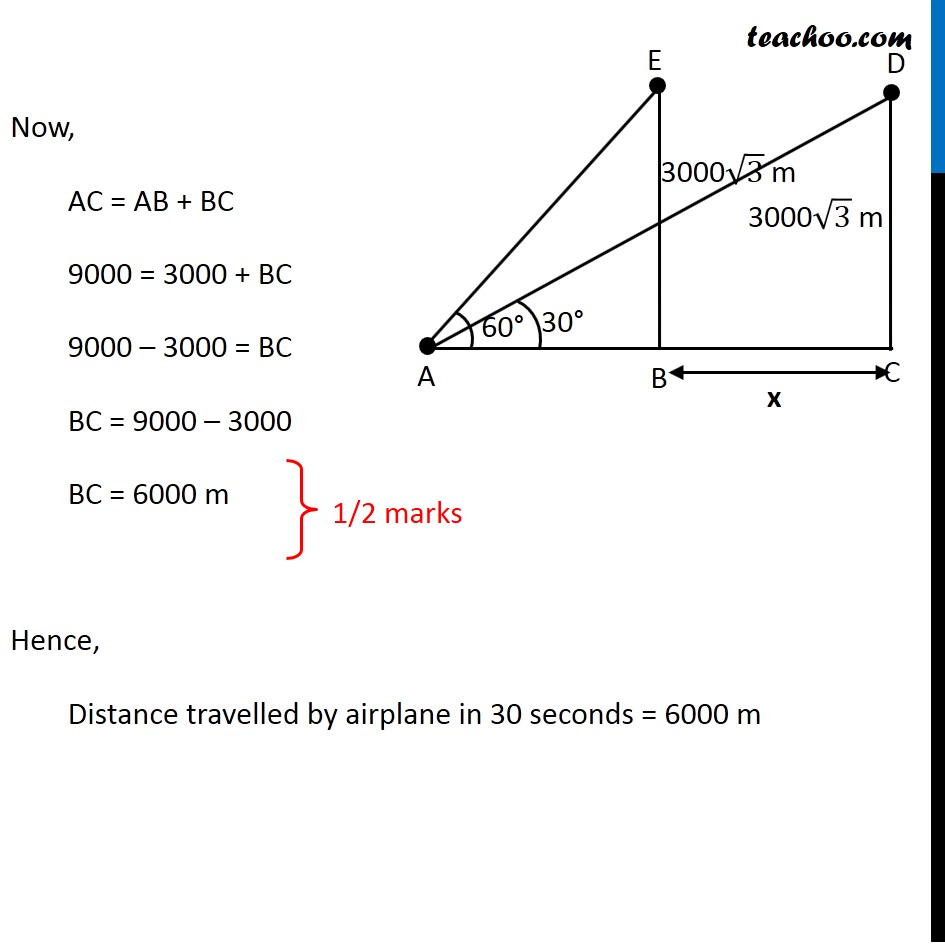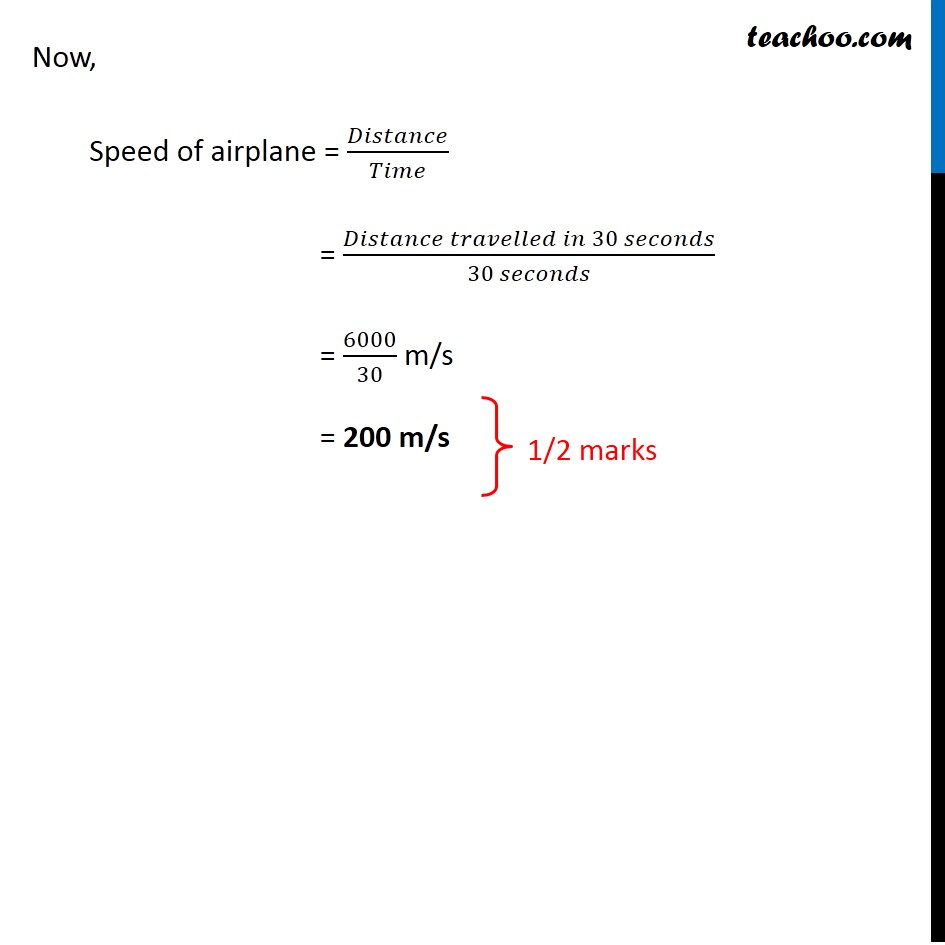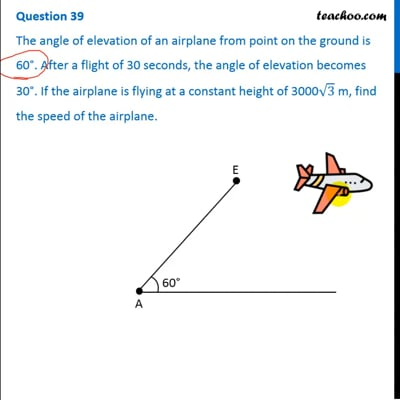This video is only available for Teachoo black users

### Transcript

Question 39 The angle of elevation of an airplane from point on the ground is 60°. After a flight of 30 seconds, the angle of elevation becomes 30°. If the airplane is flying at a constant height of 3000√3 m, find the speed of the airplane. Let the point on ground be A Since airplane is flying at height of 3000√3 m CD = BE = 3000√3 m We need to find speed of airplane To find speed, we need to distance travelled And distance travelled by airplane in 30 seconds is BC So, we need to find BC Let BC = x Here, ∠ ABE = 90° & ∠ ACD = 90° In right angle triangle ABE tan A = (𝑆𝑖𝑑𝑒 𝑜𝑝𝑝𝑜𝑠𝑖𝑡𝑒 𝑡𝑜 𝑎𝑛𝑔𝑙𝑒" " 𝐴)/(𝑆𝑖𝑑𝑒 𝑎𝑑𝑗𝑎𝑐𝑒𝑛𝑡 𝑡𝑜 𝑎𝑛𝑔𝑙𝑒" " 𝐴) tan A = (" " 𝐵𝐸)/𝐴𝐵 tan 60° = 𝐵𝐸/𝐴𝐵 √3 = (" " 3000√3)/𝐴𝐵 AB = 3000 m In right angle triangle ACD tan A = (𝑆𝑖𝑑𝑒 𝑜𝑝𝑝𝑜𝑠𝑖𝑡𝑒 𝑡𝑜 𝑎𝑛𝑔𝑙𝑒" " 𝐴)/(𝑆𝑖𝑑𝑒 𝑎𝑑𝑗𝑎𝑐𝑒𝑛𝑡 𝑡𝑜 𝑎𝑛𝑔𝑙𝑒" " 𝐴) tan A = 𝐶𝐷/𝐴𝐶 tan 30° = 𝐶𝐷/𝐴𝐶 1/√3 = (3000√3)/𝐴𝐶 AC = 3000√3 × √3 = 9000 m Now, AC = AB + BC 9000 = 3000 + BC 9000 – 3000 = BC BC = 9000 – 3000 BC = 6000 m Hence, Distance travelled by airplane in 30 seconds = 6000 m Now, Speed of airplane = 𝐷𝑖𝑠𝑡𝑎𝑛𝑐𝑒/𝑇𝑖𝑚𝑒 = (𝐷𝑖𝑠𝑡𝑎𝑛𝑐𝑒 𝑡𝑟𝑎𝑣𝑒𝑙𝑙𝑒𝑑 𝑖𝑛 30 𝑠𝑒𝑐𝑜𝑛𝑑𝑠)/(30 𝑠𝑒𝑐𝑜𝑛𝑑𝑠) = 6000/30 m/s = 200 m/s